# MA.912.AR.1.5Export Print
Divide polynomial expressions using long division, synthetic division or algebraic manipulation.
General Information
Subject Area: Mathematics (B.E.S.T.)
Strand: Algebraic Reasoning
Status: State Board Approved

## Benchmark Instructional Guide

• Algorithm
• Polynomial

### Vertical Alignment

Previous Benchmarks

Next Benchmarks

### Purpose and Instructional Strategies

In Algebra I, students perform operations on polynomials limited to 3 or fewer terms and divide polynomials by a monomial. In Mathematics for College Algebra, students perform operations on all polynomials.
• Instruction includes the connection to addition, subtraction and multiplication of polynomials to develop the understanding of closure, and the connection to properties of exponents.
• Instruction includes proper vocabulary and terminology like dividend, divisor and quotient.
• Instruction includes the connection to long division of whole numbers to develop the understanding that polynomial division follows a very similar procedure and that when the remainder is zero, the divisor and quotient are factors of the dividend (MTR.5.1)
• It is not the expectation of this benchmark that students perform division using a specific method or strategy. Students should be able to compare various methods and use the one most efficient depending on the task.
• Instruction includes the use of manipulatives, like algebra tiles, and various strategies, like the area model and properties of exponents.
• Area Model
• For example, when dividing 2$x$+ 7$x$ + 6 by $x$ + 2, students can create a table with the same number of rows as the number of terms in the divisor (as shown below).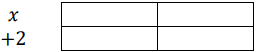• Then, students can write the first term of the dividend in the upper left rectangle. Have students determine what the length must be if the area of that rectangle (shaded yellow) is 2$x$2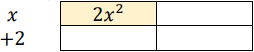• Once students determine that its length is 2$x$2 ÷ $x$ which is 2$x$, they can write the length of the rectangle.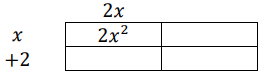• Students should be able to see that they can calculate the area of the bottom left rectangle as 2$x$(2)=4$x$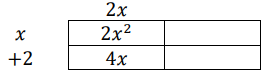• Next, students can use this information to determine the area of the upper right rectangle (shaded green). Students should notice that the second term of the dividend is 7$x$, which means that when you add the like terms diagonally you need to get 7$x$. The area of this rectangle is 7$x$ − 4$x$ = 3$x$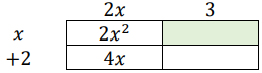• Lastly, students can calculate the length of the column, and then the area of the bottom right rectangle to determine the quotient of 2$x$2 + 7$x$ > + 6 and $x$ + 2 is 2$x$ + 3.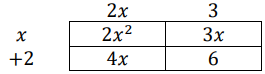### Common Misconceptions or Errors

• Students may forget to write the polynomials in standard form.
• Students may forget to write a zero when there is a missing term either in the divisor or in the dividend.
• Students may forget that synthetic division can only be used when you are dividing by a linear factor.
• When doing long division, students may add instead of subtracting the terms.
• When doing synthetic division, students may forget that the leading coefficient of the divisor should be 1.
• When doing synthetic division, students may forget to reverse the sign of the constant in the divisor.

Instructional Task 1 (MTR.3.1, MTR.5.1, MTR.7.1
• The volume of a rectangular prism is given by the polynomial 3$x$4 + 7$x$3 − 12$x$2 + 4$x$ and the area of the base is given by the polynomial 3$x$2 − 2$x$
• Part A. Find the height of the rectangular prism.
• Part B: Find the length and the width of the rectangular prism.

• Part A. Determine the quotient of 4$m$4 + 2$m$2 − 3$m$ + 2 and (2$m$ + 6).
• Part B. Compare your strategy with a partner.

### Instructional Items

Instructional Item 1
• Divide $x$4 − 2$x$3 $x$2 − 8$x$ −12 by ($x$ + 1)

Instructional Item 2
• If ($x$ − 4) is a factor of the polynomial 2$x$2 $a$$x$ − 16, what is the value of $a$

Instructional Item 3

• Determine the quotient of $x$4 + 4$x$3 − $x$ − 4 and $x$3 − 1.

*The strategies, tasks and items included in the B1G-M are examples and should not be considered comprehensive.

## Related Courses

This benchmark is part of these courses.
1200330: Algebra 2 (Specifically in versions: 2014 - 2015, 2015 - 2022, 2022 and beyond (current))
1200340: Algebra 2 Honors (Specifically in versions: 2014 - 2015, 2015 - 2022, 2022 and beyond (current))
1200400: Foundational Skills in Mathematics 9-12 (Specifically in versions: 2014 - 2015, 2015 - 2022, 2022 and beyond (current))
7912095: Access Algebra 2 (Specifically in versions: 2016 - 2018, 2018 - 2019, 2019 - 2022, 2022 and beyond (current))
1200710: Mathematics for College Algebra (Specifically in versions: 2022 and beyond (current))

## Related Access Points

Alternate version of this benchmark for students with significant cognitive disabilities.
MA.912.AR.1.AP.5: Divide polynomial expressions using long division, synthetic division and algebraic manipulation where the denominator is a linear expression.

## Related Resources

Vetted resources educators can use to teach the concepts and skills in this benchmark.

## Original Student Tutorial

Long Division With Polynomials:

Use long division to rewrite a rational expression of the form a(x) divided by b(x) in the form q(x) plus the quantity r(x) divided by b(x), where a(x), b(x), q(x), and r(x) are polynomials with this interactive tutorial.

Type: Original Student Tutorial

## Tutorial

Division of Polynomials:

This resource discusses dividing a polynomial by a monomial and also dividing a polynomial by a polynomial using long division.

Type: Tutorial

## Original Student Tutorials Mathematics - Grades 9-12

Long Division With Polynomials:

Use long division to rewrite a rational expression of the form a(x) divided by b(x) in the form q(x) plus the quantity r(x) divided by b(x), where a(x), b(x), q(x), and r(x) are polynomials with this interactive tutorial.

## Student Resources

Vetted resources students can use to learn the concepts and skills in this benchmark.

## Original Student Tutorial

Long Division With Polynomials:

Use long division to rewrite a rational expression of the form a(x) divided by b(x) in the form q(x) plus the quantity r(x) divided by b(x), where a(x), b(x), q(x), and r(x) are polynomials with this interactive tutorial.

Type: Original Student Tutorial

## Tutorial

Division of Polynomials:

This resource discusses dividing a polynomial by a monomial and also dividing a polynomial by a polynomial using long division.

Type: Tutorial

## Parent Resources

Vetted resources caregivers can use to help students learn the concepts and skills in this benchmark.